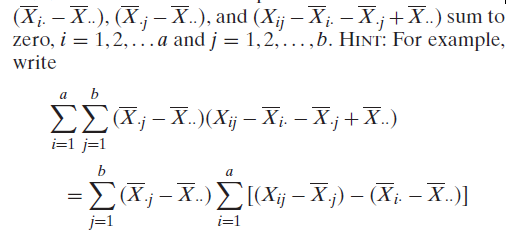×
Get Full Access to Probability And Statistical Inference - 9 Edition - Chapter 9.4 - Problem 4e
Get Full Access to Probability And Statistical Inference - 9 Edition - Chapter 9.4 - Problem 4e

×

# (In some of the exercises that follow, we must | Ch 9.4 - 4EISBN: 9780321923271 41

## Solution for problem 4E Chapter 9.4

Probability and Statistical Inference | 9th Edition

• Textbook Solutions
• 2901 Step-by-step solutions solved by professors and subject experts
• Get 24/7 help from StudySoup virtual teaching assistantsProbability and Statistical Inference | 9th Edition

4 5 1 309 Reviews
31
2
Problem 4E

Show that the cross-product terms formed fromand sum each term in the inner summation, as grouped here, to get zero.

Step-by-Step Solution:
Step 1 of 3

Personal Finance Time Value of Money ­ $1 today is worth more than$1 in the future 3 main reasons to take money today (RIO) ­ Risk: may not receive money ­ Inflation: increase in general price level ­ Opportunity cost: can do things with \$1 today Types of Problems ­ Future Value (lump sum) ­ Present Value (lump sum) ­ Annuities and loan payments o Constant streams of cash flow (per month, per year) ­ Future value of Annuities ­ Present value of Annuities ­ Perpetuities *Lump sum: 1­time investment of cash The Chart N= # of periods I= Interest rate PMT= payments PV= Present Value FV= Future Value END/BGN= on the calculator On TI­84 calculator­ press apps  TVM solver  Alpha enter You can als

Step 2 of 3

Step 3 of 3

##### ISBN: 9780321923271

Unlock Textbook Solution